# 题目描述（困难难度）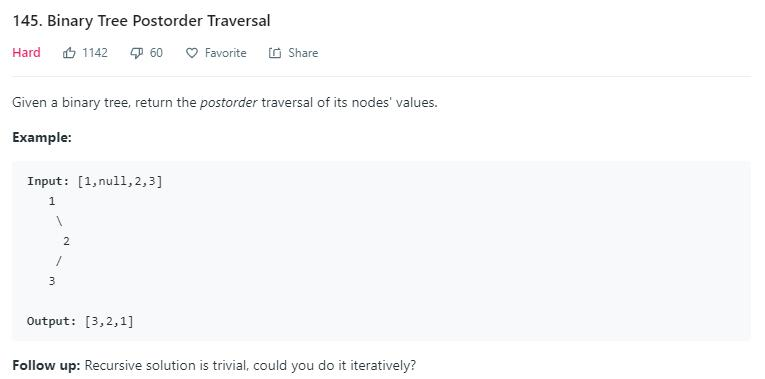# 解法一 递归

public List<Integer> postorderTraversal(TreeNode root) {
List<Integer> list = new ArrayList<>();
postorderTraversalHelper(root, list);
return list;
}

private void postorderTraversalHelper(TreeNode root, List<Integer> list) {
if (root == null) {
return;
}
postorderTraversalHelper(root.left, list);
postorderTraversalHelper(root.right, list);
}


# 解法二 栈

     1
/ \
2   3
/ \
4   5


## 1. 思想一

public List<Integer> inorderTraversal(TreeNode root) {
List<Integer> ans = new ArrayList<>();
Stack<TreeNode> stack = new Stack<>();
TreeNode cur = root;
while (cur != null || !stack.isEmpty()) {
//节点不为空一直压栈
while (cur != null) {
stack.push(cur);
cur = cur.left; //考虑左子树
}
//节点为空，就出栈
cur = stack.pop();
//当前值加入
//考虑右子树
cur = cur.right;
}
return ans;
}


public List<Integer> postorderTraversal(TreeNode root) {
List<Integer> list = new ArrayList<>();
Stack<TreeNode> stack = new Stack<>();
Set<TreeNode> set = new HashSet<>();
TreeNode cur = root;
while (cur != null || !stack.isEmpty()) {
while (cur != null && !set.contains(cur)) {
stack.push(cur);
cur = cur.left;
}
cur = stack.peek();
//右子树为空或者第二次来到这里
if (cur.right == null || set.contains(cur)) {
stack.pop();//将当前节点弹出
if (stack.isEmpty()) {
return list;
}
//转到右子树，这种情况对应于右子树为空的情况
cur = stack.peek();
cur = cur.right;
//从左子树过来，加到 set 中，转到右子树
} else {
cur = cur.right;
}
}
return list;
}


## 2. 思想二

public List<Integer> postorderTraversal(TreeNode root) {
List<Integer> list = new ArrayList<>();
Stack<TreeNode> stack = new Stack<>();
TreeNode cur = root;
TreeNode last = null;
while (cur != null || !stack.isEmpty()) {
if (cur != null) {
stack.push(cur);
cur = cur.left;
} else {
TreeNode temp = stack.peek();
//是否变到右子树
if (temp.right != null && temp.right != last) {
cur = temp.right;
} else {
last = temp;
stack.pop();
}
}
}
return list;
}


## 3. 思想三

public List<Integer> preorderTraversal(TreeNode root) {
List<Integer> list = new ArrayList<>();
if (root == null) {
return list;
}
Stack<TreeNode> stack = new Stack<>();
stack.push(root);
while (!stack.isEmpty()) {
TreeNode cur = stack.pop();
if (cur == null) {
continue;
}
stack.push(cur.right);
stack.push(cur.left);
}
return list;
}


public List<Integer> postorderTraversal(TreeNode root) {
List<Integer> list = new ArrayList<>();
if (root == null) {
return list;
}
Stack<TreeNode> stack = new Stack<>();
stack.push(root);
stack.push(root);
while (!stack.isEmpty()) {
TreeNode cur = stack.pop();
if (cur == null) {
continue;
}
if (!stack.isEmpty() && cur == stack.peek()) {
stack.push(cur.right);
stack.push(cur.right);
stack.push(cur.left);
stack.push(cur.left);
} else {
}
}
return list;
}


# 解法三 转换问题

public List<Integer> preorderTraversal(TreeNode root) {
List<Integer> list = new ArrayList<>();
Stack<TreeNode> stack = new Stack<>();
TreeNode cur = root;
while (cur != null || !stack.isEmpty()) {
if (cur != null) {
stack.push(cur);
cur = cur.left; //考虑左子树
}else {
//节点为空，就出栈
cur = stack.pop();
//考虑右子树
cur = cur.right;
}
}
return list;
}


public List<Integer> postorderTraversal2(TreeNode root) {
List<Integer> list = new ArrayList<>();
Stack<TreeNode> stack = new Stack<>();
TreeNode cur = root;
while (cur != null || !stack.isEmpty()) {
if (cur != null) {
stack.push(cur);
cur = cur.right; // 考虑左子树
} else {
// 节点为空，就出栈
cur = stack.pop();
// 考虑右子树
cur = cur.left;
}
}
Collections.reverse(list);
return list;
}


public List<Integer> preorderTraversal(TreeNode root) {
List<Integer> list = new ArrayList<>();
TreeNode cur = root;
while (cur != null) {
//情况 1
if (cur.left == null) {
cur = cur.right;
} else {
//找左子树最右边的节点
TreeNode pre = cur.left;
while (pre.right != null && pre.right != cur) {
pre = pre.right;
}
//情况 2.1
if (pre.right == null) {
pre.right = cur;
cur = cur.left;
}
//情况 2.2
if (pre.right == cur) {
pre.right = null; //这里可以恢复为 null
cur = cur.right;
}
}
}
return list;
}


public List<Integer> postorderTraversal(TreeNode root) {
List<Integer> list = new ArrayList<>();
TreeNode cur = root;
while (cur != null) {
if (cur.right == null) {
cur = cur.left;
} else {
TreeNode pre = cur.right;
while (pre.left != null && pre.left != cur) {
pre = pre.left;
}
if (pre.left == null) {
pre.left = cur;
cur = cur.right;
}
if (pre.left == cur) {
pre.left = null; // 这里可以恢复为 null
cur = cur.left;
}
}
}
Collections.reverse(list);

return list;
}


# 解法四 Morris Traversal

94 题 中序遍历中对 Morris 遍历有详细的介绍，我先贴过来。

1、cur.leftnull，保存 cur 的值，更新 cur = cur.right

2、cur.left 不为 null，找到 cur.left 这颗子树最右边的节点记做 last

2.1 last.rightnull，那么将 last.right = cur，更新 cur = cur.left

2.2 last.right 不为 null，说明之前已经访问过，第二次来到这里，表明当前子树遍历完成，保存 cur 的值，更新 cur = cur.right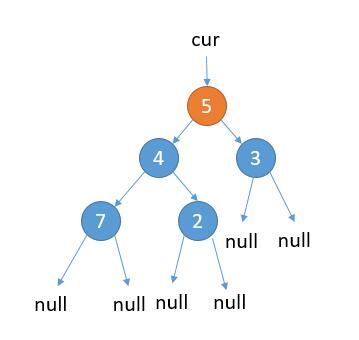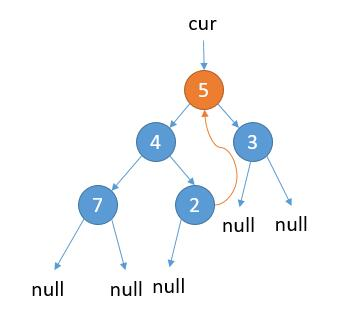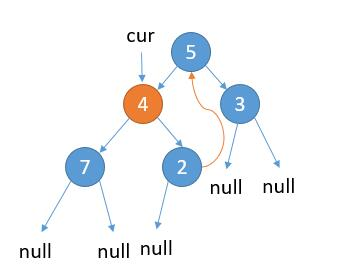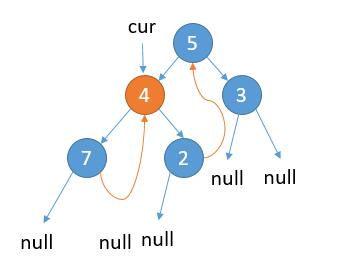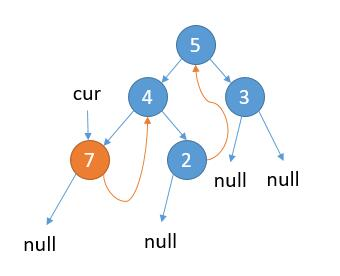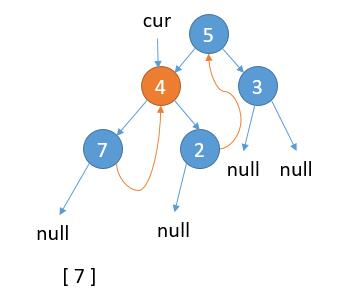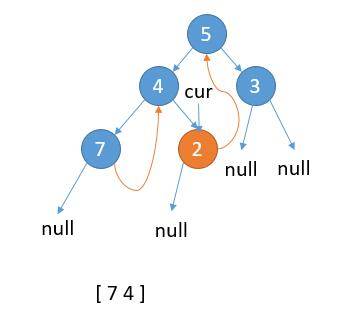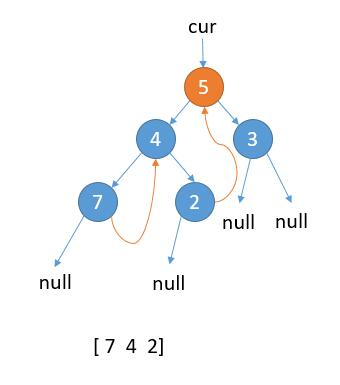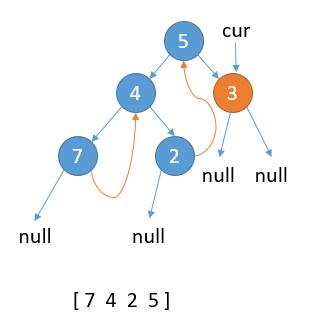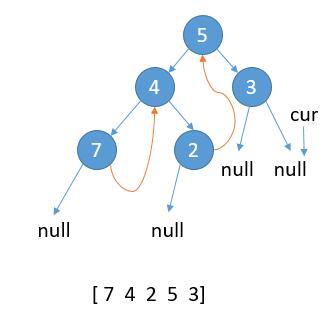cur 指向 null，结束遍历。

1、cur.leftnull，保存 cur 的值，更新 cur = cur.right

2、cur.left 不为 null，找到 cur.left 这颗子树最右边的节点记做 last

2.1 last.rightnull，那么将 last.right = cur，更新 cur = cur.left

2.2 last.right 不为 null，说明之前已经访问过，第二次来到这里，表明当前子树遍历完成，保存 cur 的值，更新 cur = cur.right

public List<Integer> inorderTraversal(TreeNode root) {
List<Integer> ans = new ArrayList<>();
TreeNode cur = root;
while (cur != null) {
//情况 1
if (cur.left == null) {
cur = cur.right;
} else {
//找左子树最右边的节点
TreeNode pre = cur.left;
while (pre.right != null && pre.right != cur) {
pre = pre.right;
}
//情况 2.1
if (pre.right == null) {
pre.right = cur;
cur = cur.left;
}
//情况 2.2
if (pre.right == cur) {
pre.right = null; //这里可以恢复为 null
cur = cur.right;
}
}
}
return ans;
}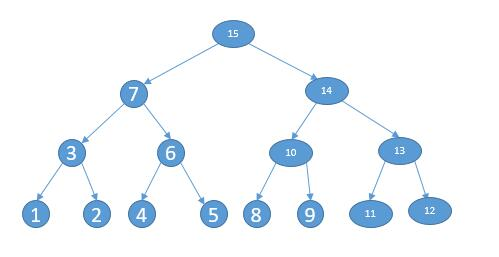先访问 15, 7, 3, 1 然后往回走
3 第二次访问，将它的左节点逆序加入到 list 中
list = 

7 第二次访问，将它的左节点，左节点的右节点逆序加入到 list 中
list = [1 2 3]

6 第二次访问, 将它的左节点逆序加入到 list 中
list = [1 2 3 4]

15 第二次访问, 将它的左节点, 左节点的右节点, 左节点的右节点的右节点逆序加入到 list 中
list = [1 2 3 4 5 6 7]

10 第二次访问，将它的左节点逆序加入到 list 中
list = [1 2 3 4 5 6 7 8]

14 第二次访问，将它的左节点，左节点的右节点逆序加入到 list 中
list = [1 2 3 4 5 6 7 8 9 10]

13 第二次访问, 将它的左节点逆序加入到 list 中
list = [1 2 3 4 5 6 7 8 9 10 11]

list = [1 2 3 4 5 6 7 8 9 10 11 12 13 14 15]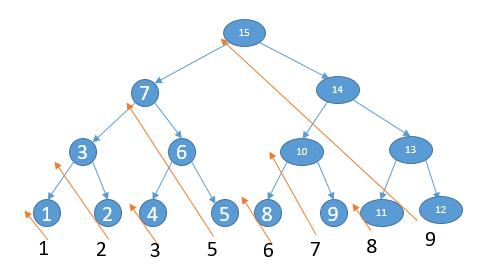public List<Integer> postorderTraversal(TreeNode root) {
List<Integer> list = new ArrayList<>();
TreeNode cur = root;
while (cur != null) {
// 情况 1
if (cur.left == null) {
cur = cur.right;
} else {
// 找左子树最右边的节点
TreeNode pre = cur.left;
while (pre.right != null && pre.right != cur) {
pre = pre.right;
}
// 情况 2.1
if (pre.right == null) {
pre.right = cur;
cur = cur.left;
}
// 情况 2.2,第二次遍历节点
if (pre.right == cur) {
pre.right = null; // 这里可以恢复为 null
//逆序
//加入到 list 中，并且把逆序还原
cur = cur.right;
}
}
}
return list;
}

return null;
}

tail.right = null;

}

return tail;
}

private TreeNode reversList(TreeNode head, List<Integer> list) {
return null;
}
tail.right = null;